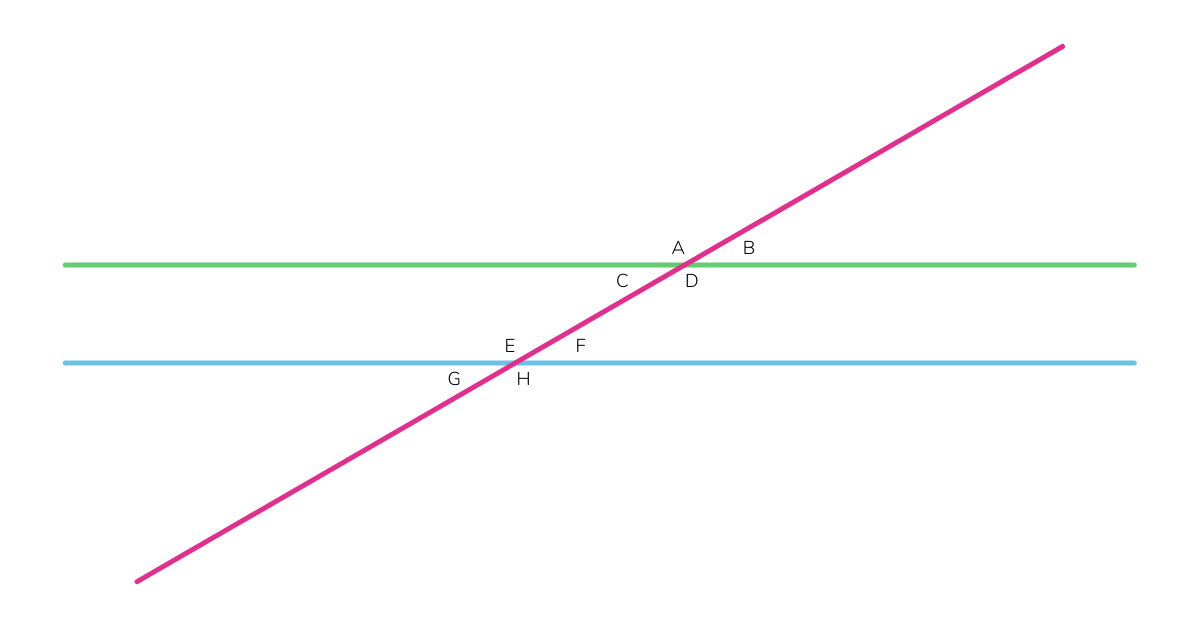Hunter's Woods PH

# Two Parallel Lines Crossed by a Transversal (Montessori Geometry)

## A quick lesson and worksheet on two parallel lines crossed by a transversal and the resulting congruent and supplementary angles.

This lesson is a continuation of Two Straight Lines Crossed by a Transversal. If you haven’t checked out that presentation yet, please do, so you can get to know the terms (especially the names of angles) that we will be using here.

When two straight parallel lines are crossed by a transversal:

• Alternate angles are congruent
• Corresponding angles are congruent
• Consecutive angles are supplementary (add up to 180°)

• Vertical or opposite angles are congruentIn the example above, the following angles are congruent:

• A, D, E and H = all 30°
• B, C, F and G = all 150°

Specifically:

• A and D are vertical angles and therefore congruent (30°).
• A and E are corresponding angles and therefore congruent (30°).
• A and H are alternate exterior angles and therefore congruent (30°).
• D and E are alternate interior angles and therefore congruent (30°).
• D and H are corresponding angles and therefore congruent (30°).
• E and H are vertical angles and therefore congruent (30°).

Likewise:

• B and C are vertical angles and therefore congruent (150°).
• B and F are corresponding angles and therefore congruent (150°).
• B and G are alternate exterior angles and therefore congruent (150°).
• C and F are alternate interior angles and therefore congruent (150°).
• C and G are corresponding angles and therefore congruent (150°).
• F and G are vertical angles and therefore congruent (150°).

## Geometry Worksheet: Two Parallel Lines Crossed by a Transversal

You can download a printable PDF copy of this worksheet here: Two Parallel Lines Crossed by a Transversal (PDF)

Note: you can reduce the size of the worksheet by zooming out your browser screen. For Windows users, scroll down the mouse wheel while pressing the Ctrl key in your keyboard.